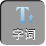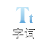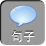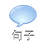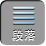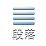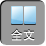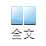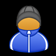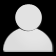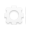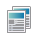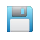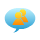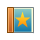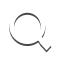-AA+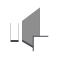1、先化简，再求值：（ 4 x -2 y-[ -2x-y）+（ 2 x+ y）] -4 x，其中 x= 0y= -3.

x= 0y= -3时，原式= -5×（ -3）= 15.

2、已知 a+ b= -2ab= 3，求 2[ ab+（ -3 a）] -32 b-ab）的值

a+ b= -2ab= 3代入，得原式= 5 ab -6a+ b）= 27.

3、有一道化简求值题：“当 a= -2b= -3时，求（ 3 a 2 b -2 ab-ab -4 a 2）+（ 3 ab-a 2 b）的值．”小芳做题时，把“ a= -2”错抄成了“ a= 2”，但她的计算结果也是正确的，请你解释一下原因？

4、在沙坪坝住房小区建设中，为了提高业主的宜居环境，某小区规划修建一个广场（平面图形如图所示）

1）用含 mn的代数式表示该广场的面积 S

2）若 mn满足（ m6 2+| n5|= 0，求出该广场的面积．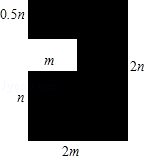2）利用非负数的性质求出 mn的值，代入 S中计算即可得到结果．

2）因为（ m6 2+| n5|= 0

1．先化简，再求值．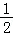x2x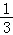y 2）+（﹣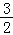x+y 2），其中 x=﹣ 2y=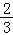2．先化简，再求值：（ 3 x 2xy+ 7）﹣（ 5 xy4 x 2+ 7），其中 xy满足（ x2 2+| 3 y1|= 0

1.解：原式=x2 x+y 2x+y 2=﹣ 3 x+ y 2.

x=﹣ 2y=时，原式=﹣ 3×（﹣ 2）+（2= 6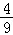2解：原式= 3 x 2xy+ 75 xy+ 4 x 27= 7 x 26 xy.